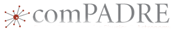# Activity: Identifying a solid using densityThis material was originally developed through comPADRE
as part of its collaboration with the SERC Pedagogic Service.

## Summary

Students will measure the length, diameter, and mass of twelve cylinders made of the same element. The first set of measurements are made with a standard metric ruler and 0.1g balance. The measurements are then repeated using a Vernier caliper and a 0.001g balance. Both sets of data a plotted to demonstrate the effect on uncertainty when more precise measuring equipment is used.

Effective measurement techniques include the concept of measurement uncertainty. Students may make erroneous conclusions analyzing data using measurements that do not include the uncertainty of the measurement. In this lab, students determine a density range for a metal and identify the material based on this range.

## Learning Goals

Students will:

• make measurements using uncertainty notation. The expression of the uncertainty depends on the the quality of the measuring device.
• demonstrate that more precise measuring tools decreases uncertainty and allows for greater confidence in conclusions drawn from the data.
• determine the probable identity of a solid based on its calculated density.

In the process of attaining these goals, the students will use a spreadsheet and graphing software to analyze data.

## Context for Use

Class size: 20-40 students

Type of activity: Lab

Time requirement: 2-3 (45 minute) classes

Special equipment: Density cylinder sets, Graphing software, 0.1g and 0.001g electronic balances, Vernier caliper

Prerequisite concepts: Density, graphing linear relationships

Course applicability: The activity is designed to introduce students to using uncertainty in lab measurements.

Adaptability: The activity can be adapted to any lab situation in which measurements are being made. The concepts are particularly useful for labs in which slight differences in measured values can make conclusions difficult to understand.

## Description and Teaching Materials

Microsoft Word version Measuring density with (un)certainty (Microsoft Word 345kB Aug31 09)

## Teaching Notes and Tips

This lab requires the students to use the following features of a spreadsheet:

• using a formula to calculate a value in a cell from data in other cells.
• "stretch" a formula over multiple cells to perform repeated calculations.

This lab requires students to use the following features of graphing software:

• create a "best fit" line from entered data.
• determine the slope of the "best fit" line.

This lab requires students to understand the following measurement concepts:

• all measurements have uncertainty.
• uncertainty results in a value range for all measurements.

Depending of the skill of the students, you may have to demonstrate these things before or during the lab.

Note that this activity asks students to plot the density of a substance as a function of mass and volume. This plot should yield a linear relationship, the slope of which is the density of the substance.

Another valid and useful approach would be to measure the mass and volume of various sized samples and determine the density of each, as well as the uncertainty of each density measurement. This way students would see the relative uncertainty of the measurement decrease as they measured larger material samples.

## Assessment

The most basic assessment is whether or not the students correctly identify the material based on the density range they calculate. Students may also be assessed using the questions at the end of the lab that probe their understanding of how using more precise measuring tools reduces uncertainty and allows for more specific conclusions from measured data.